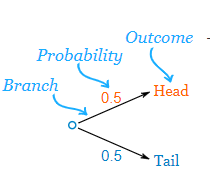# Tree Diagram Worksheets

What Are Tree Diagrams Used For? Statistics consist of different tools and methods which allow us to perform different forecasting procedures. Among those methods, the use of tree diagrams is extensive in present complex calculations. By definition, a tree diagram is just a way to represent a sequence of events. They are specifically preferable in probability since they record all the possible outcomes in a concise and comprehensive manner. Moreover, it helps in uncomplicating probabilities, which are quite hard at times, and often it is hard to figure out what to do. Let us consider the example of a simple coin toss. In this scenario, there are two branches (heads or tails). The probability of each branch is written on the branch itself. The outcome is written at the end of the branch. Look at the tree diagram below.• ### Basic Lesson

Using a snap shot of random data, students learn to read and interpret tree diagrams. A tree diagram is a way to list all possible outcomes of an event. Show the sample space for tossing one penny and rolling one die.

• ### Intermediate Lesson

Students begin to deploy and interpret tree diagrams. Bag A contains three red marbles and four blue marbles. Bag B contains five red marbles and three blue marbles. A marble is taken from each bag in turn.

• ### Independent Practice 1

Students create 20 tree diagrams. Example: Each spinner is spun once. How many outcomes are possible if spinner 1 is half blue and half orange, and spinner 2 has three equal sections labeled A, B and C. Draw a tree diagram.

• ### Independent Practice 2

Students create another 20 tree diagrams.

• ### Homework Worksheet

Offers 12 questions for home and provides an example.

• ### Skill Quiz

Students use a series of tree diagrams to answer 10 quiz questions.

• ### Homework and Quiz Answer Key

Answers for the homework and quiz.